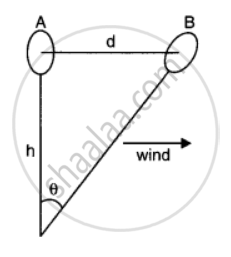# Think of Ways by Which You Can Estimate the Following (Where an Estimate is Difficult to Obtain, Try to Get an Upper Bound on the Quantity) the Wind Speed During a Storm - Physics

#### Question

Just as precise measurements are necessary in science, it is equally important to be able to make rough estimates of quantities using rudimentary ideas and common observations. Think of ways by which you can estimate the following (where an estimate is difficult to obtain, try to get an upper bound on the quantity):-

the wind speed during a storm

#### Solution 1

Wind speed during a storm can be measured by an anemometer. As wind blows, it rotates. The rotation made by the anemometer in one second gives the value of wind speed.

#### Solution 2

Wind speed can be estimated by floating a gas-filled balloon in air at a known height h. When there is no wind, the balloon is at A. Suppose the wind starts blowing to the right such that the balloon drifts to position B in 1 second.

Now, AB = d = hθ.The value of d directly gives the wind speed.

Is there an error in this question or solution?

#### APPEARS IN

NCERT Solution for Physics Textbook for Class 11 (2018 (Latest))
Chapter 2: Units and Measurements
Q: 22.3 | Page no. 37

#### Video TutorialsVIEW ALL 

Think of Ways by Which You Can Estimate the Following (Where an Estimate is Difficult to Obtain, Try to Get an Upper Bound on the Quantity) the Wind Speed During a Storm Concept: Significant Figures.